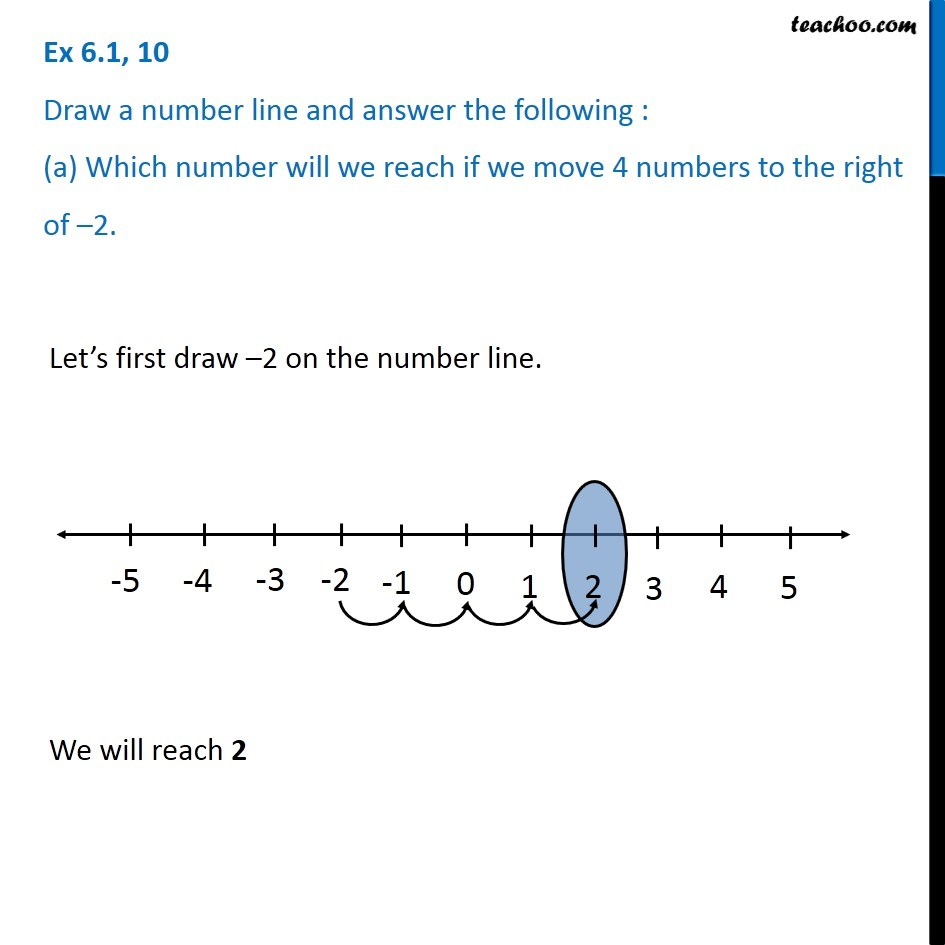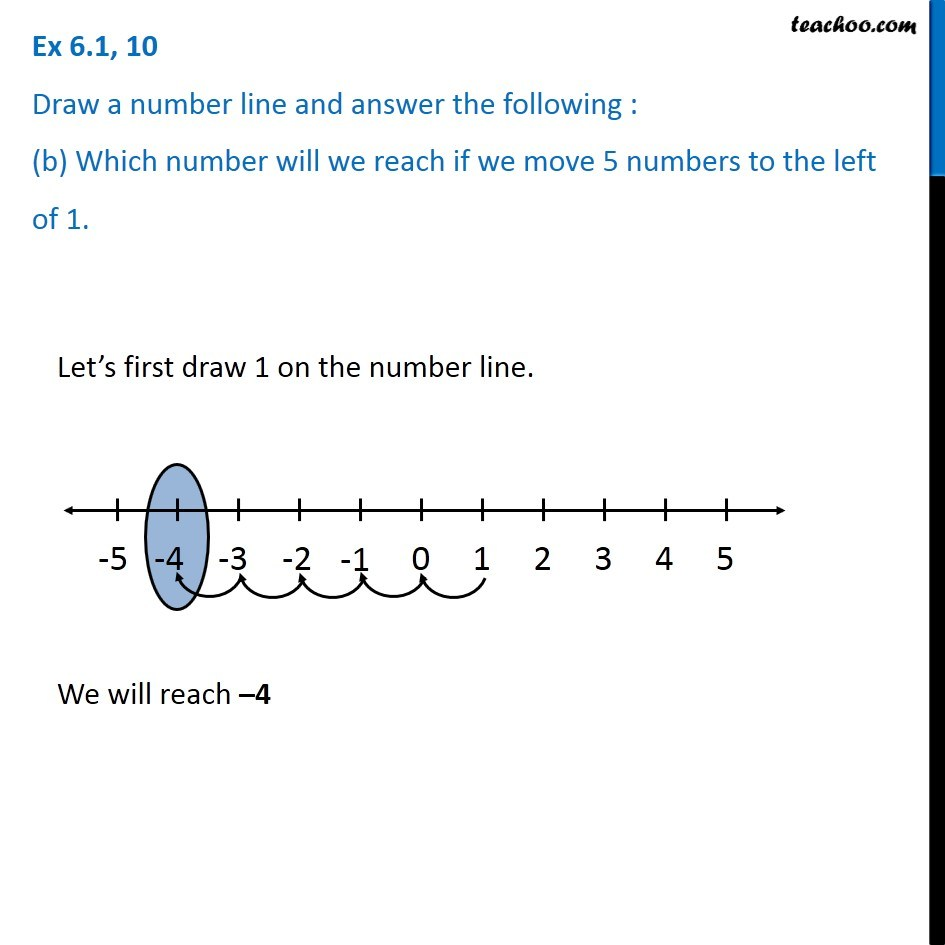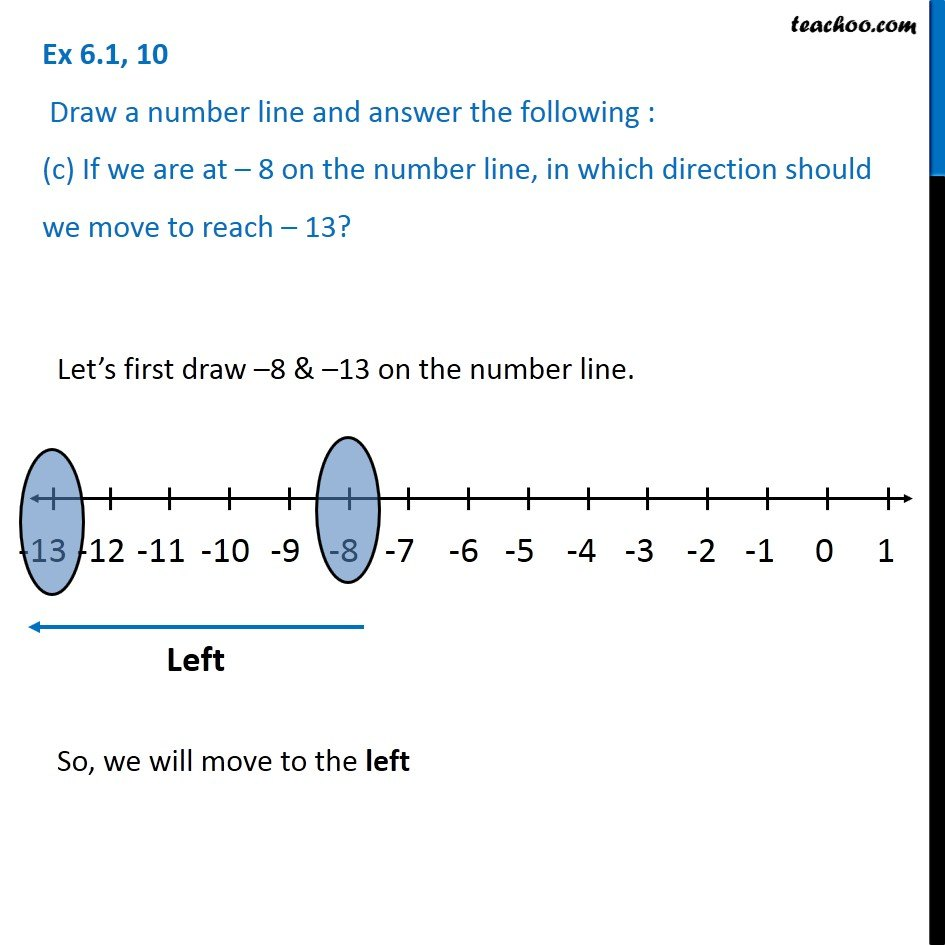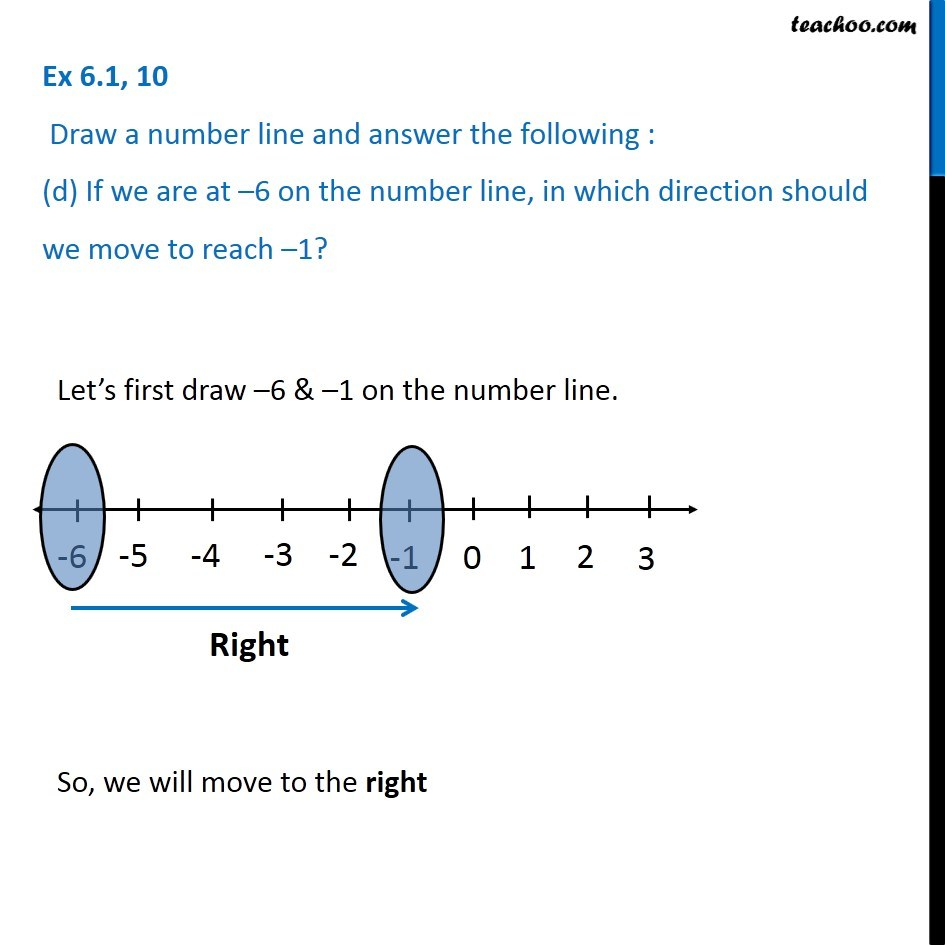Subscribe to our Youtube Channel - https://you.tube/teachoo

1. Chapter 6 Class 6 Integers
2. Serial order wise
3. Ex 6.1

Transcript

Ex 6.1, 10 Draw a number line and answer the following : (a) Which number will we reach if we move 4 numbers to the right of 2. Let s first draw 2 on the number line. We will reach 2 Ex 6.1, 10 Draw a number line and answer the following : (b) Which number will we reach if we move 5 numbers to the left of 1.Let s first draw 1 on the number line. We will reach 4 Ex 6.1, 10 Draw a number line and answer the following : (c) If we are at 8 on the number line, in which direction should we move to reach 13? Let s first draw 8 & 13 on the number line. So, we will move to the left Ex 6.1, 10 Draw a number line and answer the following : (d) If we are at 6 on the number line, in which direction should we move to reach 1? Let s first draw 6 & 1 on the number line. So, we will move to the right

Ex 6.1

Chapter 6 Class 6 Integers
Serial order wise